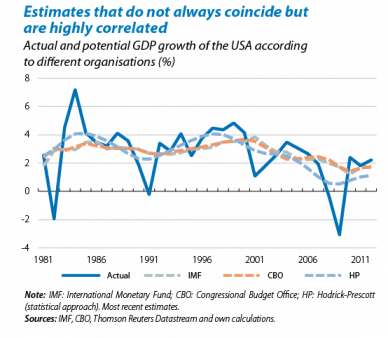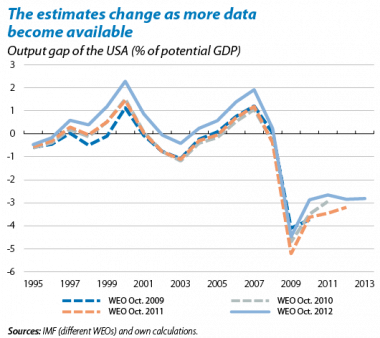# May 2013 | No. 368

## How is potential GDP calculated?

Content available in

Both the GDP and employment of the countries in the epicentre of the crisis have been significantly hit. Yet the damage could be greater if the crisis hindered their production capacity or growth in the long term which, in economics jargon, is known as potential GDP and potential growth, respectively. How can we verify if this is the case? Potential GDP is an unobserved variable that has important policy implications so we have no choice but to estimate it.(1) However, as with all estimates, there is room for error and also for discrepancy since the outcome will largely depend on the methodology used.

Economists and analysts have drawn up different strategies to calculate potential output. These can be classified into three broad categories: methods that determine the trend in the cyclical part of GDP using statistical techniques; structural methods based on relations resulting from economic theory; and mixed methods that combine statistical procedures to filter the trend with elements based on economic theory. Whether one or the other is used depends primarily on data availability. In particular, most statistical methods are not very data-intensive since they only require output data to calculate the potential figure. On the other hand, structural methods, as they are based on a theoretical model that relates output to other variables, require a large amount of information that is not always available. For this reason, the International Monetary Fund (IMF), for example, does not define a standard methodology to calculate potential output but prefers to use the one that best suits the situation and availability of data for the country in question.

One of the main statistical methods is the Hodrick-Prescott (HP) filter which extracts a trend that is reasonably in line with the evolution observed in output and is «smooth»; i.e. it doesn't change too much from year to year. This data smoothing is based on the premise that variability in potential output, in the short term, cannot be excessive given that, on the one hand, the availability of basic production factors (labour and capital) is relatively inertial and, on the other, new technologies are slow to spread. The method's simplicity and its few requirements in terms of data (it only needs a sufficiently long series of GDP figures) are the advantages of the HP filter, as it can be applied to most countries. However, because of how it is constructed, the estimated potential output can be reasonably well adjusted to the middle years of the sample but not to the two extremes. This makes it difficult to use for contemporary estimates of potential output. Another drawback is the discretion allowed to the analyst in terms of the degree of «smoothing» carried out on the estimated trend.

Given these drawbacks, and although the HP filter is the most commonly used, the main international bodies resort to structural methods whenever possible.(2) These methods are based on more or less complex versions of the production function to produce a theory-based relation between a country's output and its production factors and technology. In practice, first the potential of the production factors and technology is calculated; these are then incorporated within the chosen production function and the result is the estimated potential GDP.

The wide range of options, both in terms of production function and possible strategies used to estimate the potential of production factors and technology, means that the resulting estimates can vary greatly. By way of example, the previous graph illustrates how the estimates for the potential output of the USA, calculated by the IMF and CBO, do not coincide even though both institutions use a methodology based on the production function. This discrepancy is even greater when these series are compared with the potential output obtained using an HP filter. The estimates also vary over time, as new data are included in the estimate.

In addition to greater variability in the resulting estimate, the need to first calculate the potential of labour, capital and technology also makes this method more complicated in technical terms. For example, the calculation of the potential labour force or human capital depends crucially on initial assumptions and on how the estimates are calculated regarding future demographic trends, the activity rate in the labour market (labour force), labour legislation and educational policy, among other elements.

Similarly, calculating the potential value of capital services is particularly complex, due fundamentally to the difficulty posed by the heterogeneous composition of the capital factor (from factories to computers and patents). By way of example: one of the customary ways of calculating capital services is the perpetual inventory method, which estimates the existing capital stock based on past investment flows and the rate of depreciation. However, how to choose the depreciation rate? It seems logical to suppose that a computer depreciates more quickly than a building but, by how much?

Regarding technology, most organisations calculate its contribution as a residual (known in academic literature as the Solow residual); i.e. the part of economic growth that cannot be explained either by physical capital accumulation or a change in the labour factor. Using the historical technology series estimated as this residual, their potential is normally calculated via statistical methods such as the HP filter applied to the productivity series.

The structural approach is widely used in spite of its greater requirements in terms of data, its technical difficulty and disparity of results. Its main appeal lies in the fact that, by using the production function, the sources of any improvement or deterioration in economic growth can be well and easily identified, thereby resulting in well-substantiated recommendations.

Halfway between the oversimplification of statistical methods and the high technical and data requirements of structural methods are the mixed methods which, as their name suggests, combine the features of the other two approaches.(3) More specifically, they complement the statistical filters with relations based on economic theory. As a result of how they are constructed, these methods bring together some of the advantages and disadvantages of the two previous categories. For example, although the need for data is less when they include theory-based relations, the relative weight given to this economic information is, once again, arbitrary and depends on each analyst.

In short, there is no such thing as the perfect method so that, whatever approach is chosen, it is essential to maintain a critical view and be aware both of its qualities and its drawbacks. It is therefore no surprise that a calculation error, no matter how small, could have significant repercussions.(4)